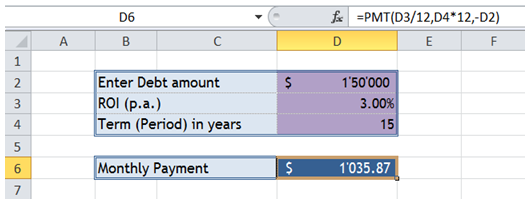## Calculate loan amount based on interest rate and period in Excel

To do it in Excel, here is the answer:

a) Enter the formula =PMT(D3/12,D4*12,-D2) where D2 corresponds to "Debt Amount", D3 corresponds to Rate of Interest per annum and D4 corresponds to the period in years.